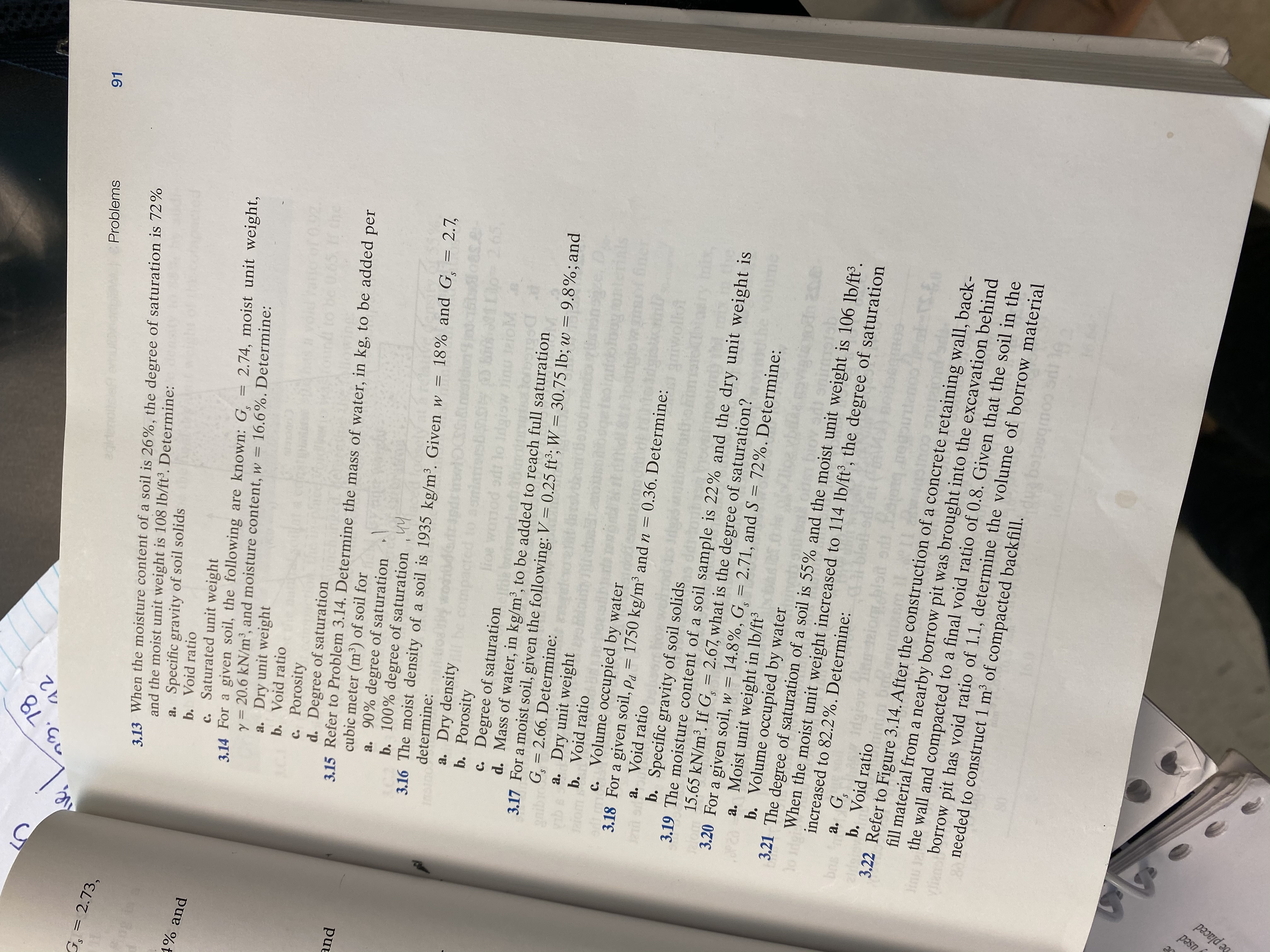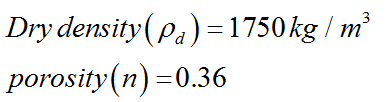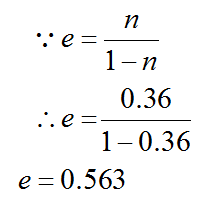# つ3.781291ProblemsG,= 2.73,3.13 When the moisture content of a soil is 26%, the degree of saturation is 72%and the moist unit weight is 108 lb/ft³. Determine:a. Specific gravity of soil solidsb. Void ratioc. Saturated unit weight3.14 For a given soil, the following are known: G.y = 20.6 kN/m', and moisture content, w = 16.6%. Determine:a. Dry unit weightb. Void ratio1% and2.74, moist unit weight,of 0.92Chec. Porosityd. Degree of saturation3.15 Refer to Problem 3.14. Determine the mass of water, in kg, to be added percubic meter (m³) of soil fora. 90% degree of saturationb. 100% degree of saturation ,443.16 The moist density of a soil is 1935 kg/m³. Given w = 18% and G, = 2.7,determine:anda. Dry densityb. Porosityc. Degree of saturationd. Mass of water, in kg/m³, to be added to reach full saturation3.17 For a moist soil, given the following: V = 0.25 ft³; W =G = 2.66. Determine:a. Dry unit weightb. Void ratio2.65ed tolioe30.75 lb; w = 9.8%; andS.c. Volume occupied by water3.18 For a given soil, p, = 1750 kg/m³ and n =a. Void ratio0.36. Determine:b. Specific gravity of soil solids3.19 The moisture content of a soil sample is 22% and the dry unit weight is15.65 kN/m³. If G = 2.67, what is the degree of saturation?3.20 For a given soil, w = 14.8%, G, = 2.71, and S = 72%. Determine:a. Moist unit weight in lb/ft³b. Volume occupied by water3.21 The degree of saturation of a soil is 55% and the moist unit weight is 106 lb/ft³.%3DWhen the moist unit weight increased to 114 lb/ft°, the degree of saturationloIdgincreased to 82.2%. Determine:a. G.b. Void ratio3.22 Refer to Figure 3.14. After the construction of a concrete retaining wall, back-fill material from a nearby borrow pit was brought into the excavation behindbnsinu the wall and compacted to a final void ratio of 0.8. Given that the soil in theborrow pit has void ratio of 1.1, determine the volume of borrow materialपरदान2 needed to construct 1 m2 of compacted backfill.pasneplaced

Question
9 views

How to solve 3.18help_outlineImage Transcriptioncloseつ3.78 12 91 Problems G,= 2.73, 3.13 When the moisture content of a soil is 26%, the degree of saturation is 72% and the moist unit weight is 108 lb/ft³. Determine: a. Specific gravity of soil solids b. Void ratio c. Saturated unit weight 3.14 For a given soil, the following are known: G. y = 20.6 kN/m', and moisture content, w = 16.6%. Determine: a. Dry unit weight b. Void ratio 1% and 2.74, moist unit weight, of 0.92 Che c. Porosity d. Degree of saturation 3.15 Refer to Problem 3.14. Determine the mass of water, in kg, to be added per cubic meter (m³) of soil for a. 90% degree of saturation b. 100% degree of saturation ,44 3.16 The moist density of a soil is 1935 kg/m³. Given w = 18% and G, = 2.7, determine: and a. Dry density b. Porosity c. Degree of saturation d. Mass of water, in kg/m³, to be added to reach full saturation 3.17 For a moist soil, given the following: V = 0.25 ft³; W = G = 2.66. Determine: a. Dry unit weight b. Void ratio 2.65 ed to lioe 30.75 lb; w = 9.8%; and S. c. Volume occupied by water 3.18 For a given soil, p, = 1750 kg/m³ and n = a. Void ratio 0.36. Determine: b. Specific gravity of soil solids 3.19 The moisture content of a soil sample is 22% and the dry unit weight is 15.65 kN/m³. If G = 2.67, what is the degree of saturation? 3.20 For a given soil, w = 14.8%, G, = 2.71, and S = 72%. Determine: a. Moist unit weight in lb/ft³ b. Volume occupied by water 3.21 The degree of saturation of a soil is 55% and the moist unit weight is 106 lb/ft³. %3D When the moist unit weight increased to 114 lb/ft°, the degree of saturation lo Idg increased to 82.2%. Determine: a. G. b. Void ratio 3.22 Refer to Figure 3.14. After the construction of a concrete retaining wall, back- fill material from a nearby borrow pit was brought into the excavation behind bns inu the wall and compacted to a final void ratio of 0.8. Given that the soil in the borrow pit has void ratio of 1.1, determine the volume of borrow material परदान 2 needed to construct 1 m2 of compacted backfill. pasn eplaced fullscreen
check_circle

Step 1

Given data:Step 2

a)

Calculate void ratio:...

### Want to see the full answer?

See Solution

#### Want to see this answer and more?

Solutions are written by subject experts who are available 24/7. Questions are typically answered within 1 hour.*

See Solution
*Response times may vary by subject and question.
Tagged in